### Home > A2C > Chapter 2 > Lesson 2.1.4 > Problem2-47

2-47.
1. Throughout this book, key problems have been selected as “checkpoints.” Each checkpoint problem is marked with an icon like the one at left. These checkpoint problems are provided so that you can check to be sure you are building algebra skills at the expected level. When you have trouble with checkpoint problems, refer to the review materials and practice problems that are available in the “Checkpoint Materials” section at the back of your book. Homework Help ✎

2. This problem is a checkpoint for using the slope-intercept form of a line to solve a system of linear equations. It will be referred to as Checkpoint 1.1. Solve the system below by graphing each line and finding the intersection. Then solve the system algebraically to check.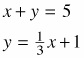2. Check your answer to part (a) by referring to the Checkpoint 1 materials located at the back of your book.

If you needed help solving this problem correctly, then you need more practice using the slope-intercept form of a line to solve a system of equations. Review the Checkpoint 1 materials and try the practice problems. Also, consider getting help outside of class time. Be sure you know how to write the equations in y-form and know how use the slope and y-intercept to draw graphs efficiently. From this point on, you will be expected to graph and solve systems like this one quickly and easily.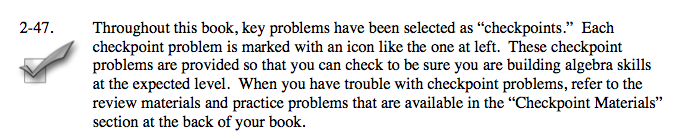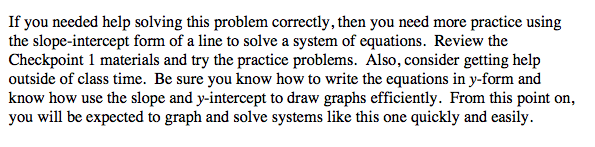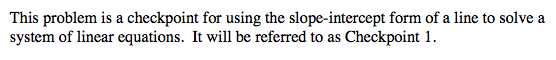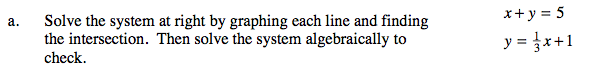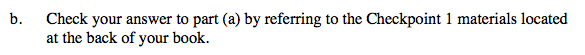Answers and extra practice are located in the back of your printed textbook or in the Reference Tab of your eBook.
If you have an eBook for A2C, login and then click the following link: Checkpoint 1 Answers and Practice